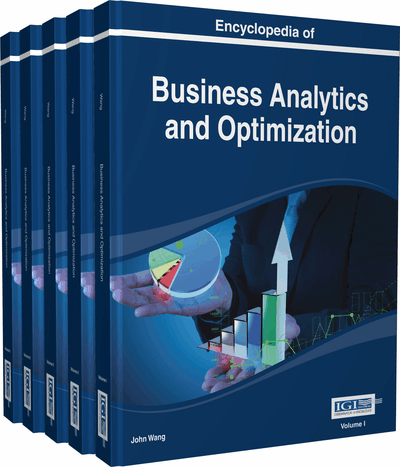# Penalized Splines with an Application in Economics

Chaojiang Wu (Drexel University, USA)
DOI: 10.4018/978-1-4666-5202-6.ch163

Top

## Background

Nonparametric smoothing or nonparametric regression is a technique for fitting curves, where the form of the curve is not predetermined but estimated through data. In its simplest form, given data pairs (,), i = 1,…, n, the classical simple linear regression model is,whereis the intercept andis the slope parameter,is error term, usually assumed to be i.i.d. N(0,σ2). In the above model, a predetermined linear functional form describes the dependence of response variable y on predictor x; the parametersandare to be estimated. However, in many cases, it is inappropriate to fit a straight line. Alternatively, response variable y takes the following form,where g(·) is an unspecified function andis again i.i.d. N(0,σ2). No parameters are explicitly present in the model. In other words, we are trying to estimate the function rather than estimating the parameters in any predetermined form.

The most popular ways of estimating g(·) are local polynomial methods (local methods) and spline methods (global methods). Some excellent references on local methods can be found in Wand and Jones (1995) and Fan and Gijbels (1996). The method introduced in this chapter (P-spline) is one type of global methods. There are generally three types of spline methods: smoothing splines, regression splines and penalized splines. Penalized splines or P-splines can be viewed as a generalization of smoothing splines with a more flexible choice of bases and penalties. Alternatively, P-splines can be viewed as least square regression splines with a roughness penalty. Some discussions on smoothing spline methods can be seen in Wahba (1990) and Eubank (1988). General discussions on penalized splines can be found in Ruppert, Wand and Carroll (2003). Applications of penalized splines have gained popularity only recently. Some examples can be found in Jarrow, Ruppert and Yu (2004) on estimating the term structure of corporate bonds, Brunauer, Lang, Wechselberger and Bienert (2010) on estimating the nonlinear effects and smooth time trend in modeling the rent in Vienna, and Çetinyürek and Lambert (2011) on estimating the survival function and hazard ratios for interval-censored data in Medicine.

## Key Terms in this Chapter

Roughness Penalty: Penalty term added to the least square objective function that controls overfitting by shrinking unnecessary knots.

Spline Basis: Piecewise polynomial functions of which any smooth function can be expressed as linear combinations.

Penalized Spline: or P-spline, is a variant of smoothing spline with more flexible choice of bases, knots and penalties. Alternatively, P-spline can be viewed as least square regression spline with a roughness penalty.

Knots: The points where piecewise polynomial functions join.

Smoothing Parameter: In P-spline set up, smoothing parameter is the only controlling parameter that controls the smoothness of the fitted curve.

Functional Coefficient Autoregressive Model: Nonlinear modeling approach to time series where the autoregressive coefficients are smooth functions.

Degree of P-Spline: The degree of polynomials.

Nonparametric Smoothing: Also sometimes called nonparametric regression, is a technique for fitting curves, where the form of the curve is not predetermined but estimated through data.

## Complete Chapter List

Search this Book:
Reset